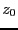Next: Full continuation procedure Up: Implementation Previous: Certified Newton   Contents

Procedure for following branches

This procedure takes as input a set ofsolutions of the system and will return points on thebranches. The branches will be followed until a given value for the parameter is reached or if Kantorovitch theorem is no more satisfied for some value of the parameter. It is implemented as:

int ALIAS_Start_Continuation(int m,int n,int NUM,
INTERVAL_MATRIX &Solutions,
INTERVAL_VECTOR (* IntervalFunction)(int,int,INTERVAL_VECTOR &),
INTERVAL_MATRIX (* IntervalHessian)(int,int,INTERVAL_VECTOR &),
double epsilon,double epsilonf,
double *z,double delta,double mindelta,
INTERVAL &Rangez,
int sens,
MATRIX &BRANCH,int *NBBRANCH)
the arguments being:
• m: number of unknowns
• n: number of equations
• NUM: the number of solutions of the system for the current value of the parameter z
• Solutions: the solutions of the system for the current value of the parameter z
• IntervalFunction: a function which return the interval vector evaluation of the equations, see note 2.3.4.3.
• IntervalGradient: a function which return the interval matrix of the jacobian of the equations, see note 2.4.2.2
• IntervalHessian: a function which return the interval matrix of the Hessian of the equations, see note 2.5.2.1
• epsilon: the maximal width of the box, see the note 2.3.4.6. If all the variable ranges have a width lower than this value and the interval evaluation of the equations contains all 0, then the set of ranges is considered to be a solution. But they will be not considered as a valid solution as they will not satisfy Kantorovitch theorem. Hence you must put here a very small value
• epsilonf: the maximal width of the equation intervals, see the note 2.3.4.6. This value will be used by the iterative Newton scheme to stop the iteration.
• z: the parameter of the system
• delta: ifis the first value of the parameter such that the system has solutions, then we will store in BRANCH the solutions for+delta,+2delta,...
• mindelta: if the algorithm is unable to find a Kantorovitch solution for the parameter value z+mindelta while a solution has been found for z, then the algorithm will assume that we are close to a singular point. Hence mindelta should have a small value
• Rangez: the range for the parameter z
• sens: either 1 or -1. If 1 the branch will be computed for increasing values of z, if -1 for decreasing values of z. This parameter should be chosen according to the largest number of solutions of the system for the lower and upper value of z
• BRANCH: the procedure will return an array of NBBRANCH lines and m+2 columns, which describe the points of the branch. In a line the first m+1 elements are the coordinates of the point and the m+2 elements is the number of the branch to which belong this point. The points are not ordered with respect to the branch number. The algorithm will take care of resizing BRANCH as needed, hence there is no need to give dimension for this parameter
• NBBRANCH: the total number of points in the array BRANCH.
The return code is:
• 1: the branches have been successfully determined
• -1, -2: the algorithm has failed to find a Kantorovitch solution for the current value of the parameter
• -3: failure in Newton method (should not occur)
• -10: for the current value of the parameter the jacobian is singular
• -20: sens is not 1 or -1
• -30: delta or mindelta is negative
Even for a negative return code the points in BRANCH are valid: the procedure will return the points that have computed on thebranches but is simply not able to determine the location of new branches that may have appeared.Next: Full continuation procedure Up: Implementation Previous: Certified Newton   Contents
Jean-Pierre Merlet 2012-12-20